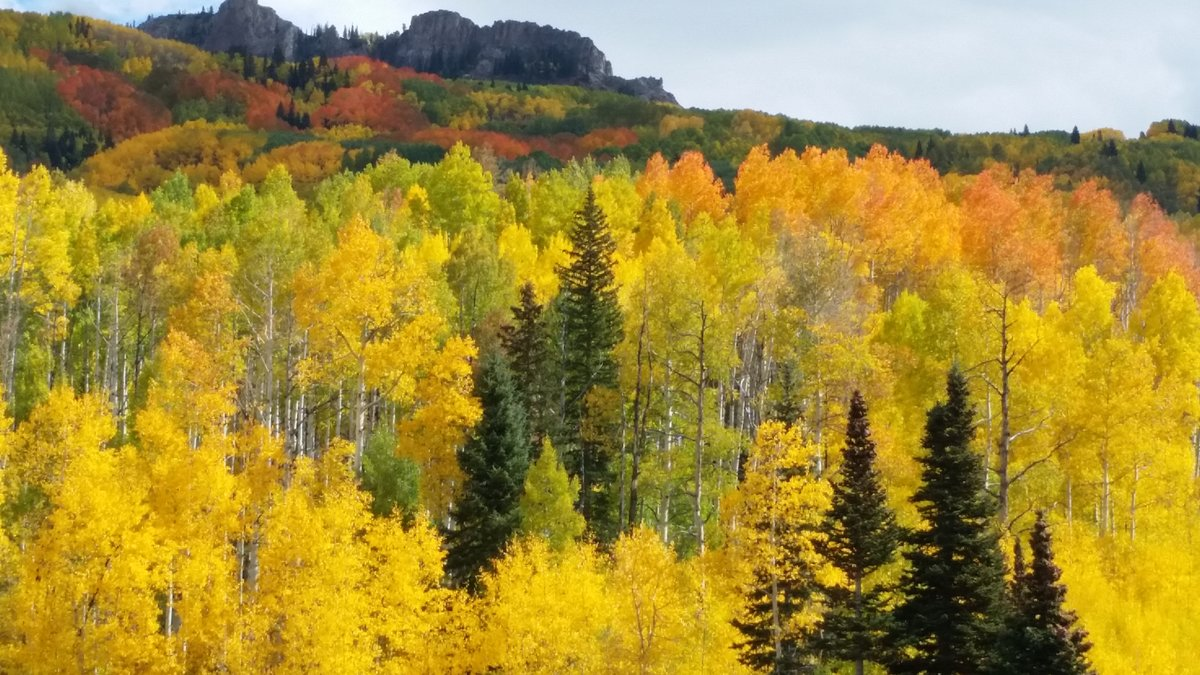# How to Do Histogram Matching Using OpenCVIn this tutorial, you will learn how to do histogram matching using OpenCV. Histogram matching (also known as histogram specification), is the transformation of an image so that its histogram matches the histogram of an image of your choice (we’ll call this image of your choice the “reference image”).

For example, consider this image below.

We want the image above to match the histogram of the reference image below.

After performing histogram matching, the output image needs to look like this:

Then, to make things interesting, we want to use this mask to mask the output image.

# Directions

Below is the source code for the program that makes everything happen. Make sure you copy and paste this code into a single Python file (mine is named histogram_matching.py). Then put that file, as well as your source, reference, and mask images all in the same directory (or folder) in your computer. Once you have done that, run the code using the following command (note: mask image is optional):

`python histogram_matching.py <source_image> <ref_image> [<mask_image>]`

For example (put this command all on one line):

`python histogram_matching.py aspens_in_fall.jpg forest_resized.jpg mask.jpg`

## Source Code

```#!/usr/bin/env python

'''
Welcome to the Histogram Matching Program!

Given a source image and a reference image, this program
returns a modified version of the source image that matches
the histogram of the reference image.

Image Requirements:
- Source image must be color.
- Reference image must be color.
- The sizes of the source image and reference image do not
have to be the same.
- The program supports an optional third image (mask) as
an argument.
- When the mask image is provided, it will be rescaled to
be the same size as the source image, and the resulting

Usage:
'''

# Python 2/3 compatibility
from __future__ import print_function

import cv2 # Import the OpenCV library
import numpy as np # Import Numpy library
import matplotlib.pyplot as plt # Import matplotlib functionality
import sys # Enables the passing of arguments

# Project: Histogram Matching Using OpenCV
# Date created: 9/27/2019
# Python version: 3.7

# Define the file name of the images
SOURCE_IMAGE = "aspens_in_fall.jpg"
REFERENCE_IMAGE = "forest_resized.jpg"
OUTPUT_IMAGE = "aspens_in_fall_forest_output"

def calculate_cdf(histogram):
"""
This method calculates the cumulative distribution function
:param array histogram: The values of the histogram
:return: normalized_cdf: The normalized cumulative distribution function
:rtype: array
"""
# Get the cumulative sum of the elements
cdf = histogram.cumsum()

# Normalize the cdf
normalized_cdf = cdf / float(cdf.max())

return normalized_cdf

def calculate_lookup(src_cdf, ref_cdf):
"""
This method creates the lookup table
:param array src_cdf: The cdf for the source image
:param array ref_cdf: The cdf for the reference image
:return: lookup_table: The lookup table
:rtype: array
"""
lookup_table = np.zeros(256)
lookup_val = 0
for src_pixel_val in range(len(src_cdf)):
lookup_val
for ref_pixel_val in range(len(ref_cdf)):
if ref_cdf[ref_pixel_val] >= src_cdf[src_pixel_val]:
lookup_val = ref_pixel_val
break
lookup_table[src_pixel_val] = lookup_val
return lookup_table

def match_histograms(src_image, ref_image):
"""
This method matches the source image histogram to the
reference signal
:param image src_image: The original source image
:param image  ref_image: The reference image
:return: image_after_matching
:rtype: image (array)
"""
# Split the images into the different color channels
# b means blue, g means green and r means red
src_b, src_g, src_r = cv2.split(src_image)
ref_b, ref_g, ref_r = cv2.split(ref_image)

# Compute the b, g, and r histograms separately
# The flatten() Numpy method returns a copy of the array c
# collapsed into one dimension.
src_hist_blue, bin_0 = np.histogram(src_b.flatten(), 256, [0,256])
src_hist_green, bin_1 = np.histogram(src_g.flatten(), 256, [0,256])
src_hist_red, bin_2 = np.histogram(src_r.flatten(), 256, [0,256])
ref_hist_blue, bin_3 = np.histogram(ref_b.flatten(), 256, [0,256])
ref_hist_green, bin_4 = np.histogram(ref_g.flatten(), 256, [0,256])
ref_hist_red, bin_5 = np.histogram(ref_r.flatten(), 256, [0,256])

# Compute the normalized cdf for the source and reference image
src_cdf_blue = calculate_cdf(src_hist_blue)
src_cdf_green = calculate_cdf(src_hist_green)
src_cdf_red = calculate_cdf(src_hist_red)
ref_cdf_blue = calculate_cdf(ref_hist_blue)
ref_cdf_green = calculate_cdf(ref_hist_green)
ref_cdf_red = calculate_cdf(ref_hist_red)

# Make a separate lookup table for each color
blue_lookup_table = calculate_lookup(src_cdf_blue, ref_cdf_blue)
green_lookup_table = calculate_lookup(src_cdf_green, ref_cdf_green)
red_lookup_table = calculate_lookup(src_cdf_red, ref_cdf_red)

# Use the lookup function to transform the colors of the original
# source image
blue_after_transform = cv2.LUT(src_b, blue_lookup_table)
green_after_transform = cv2.LUT(src_g, green_lookup_table)
red_after_transform = cv2.LUT(src_r, red_lookup_table)

# Put the image back together
image_after_matching = cv2.merge([
blue_after_transform, green_after_transform, red_after_transform])
image_after_matching = cv2.convertScaleAbs(image_after_matching)

return image_after_matching

"""
This method overlays a mask on top of an image
:param image image: The color image that you want to mask
:rtype: image (array)
"""

# Split the colors into the different color channels
blue_color, green_color, red_color = cv2.split(image)

# Resize the mask to be the same size as the source image

# Scale the color values
blue_color = blue_color.astype(int)
green_color = green_color.astype(int)
red_color = red_color.astype(int)

# Put the image back together again
merged_image = cv2.merge([blue_color, green_color, red_color])

def main():
"""
Main method of the program.
"""
start_the_program = input("Press ENTER to perform histogram matching...")

# A flag to indicate if the mask image was provided or not by the user

# Pull system arguments
try:
image_src_name = sys.argv
image_ref_name = sys.argv
except:
image_src_name = SOURCE_IMAGE
image_ref_name = REFERENCE_IMAGE

try:
except:
print("\nNote: A mask was not provided.\n")

# Load the images and store them into a variable

# Check if the images loaded properly
if image_src is None:
print('Failed to load source image file:', image_src_name)
sys.exit(1)
elif image_ref is None:
print('Failed to load reference image file:', image_ref_name)
sys.exit(1)
else:
# Do nothing
pass

# Convert the image mask to grayscale

# Calculate the matched image
output_image = match_histograms(image_src, image_ref)

# Save the output images
cv2.imwrite(OUTPUT_IMAGE, output_image)

## Display images, used for debugging
cv2.imshow('Source Image', image_src)
cv2.imshow('Reference Image', image_ref)
cv2.imshow('Output Image', output_image)